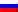# Markov process

Contenus

## Markov process

A Markov process represents all processes with random experience arguments.

A random experiment, noted E, is an experiment whose outcome is subject to chance. We denote by Ω the set of all the possible results of this experiment, and is called universe, space of possibilities or even space of states. A result of E is an element of Ω noted ω.

For example, in the coin flip game, the universe of the "flip a coin" experience is Ω = {P, F}. For the experiment "toss two coins one after the other", the universe is Ω = {PP, PF, FP, FF}.

A random event A linked to the experiment E is a subset of Ω which one can tell in the light of the experiment whether it is realized or not. In the previous example the random event “getting heads” in a coin toss can easily be observed by flipping a coin. A random event is a set and therefore has the main properties of set theory.

Elementary operations on the parts of a set:

• Intersection: the intersection of the sets A and B noted A ∩B is the set
points belonging to both A and B.
• Reunion: the reunion of two sets A and B denoted A∪B is the set of
points belonging to at least one of the two sets.
• Empty set: the empty set, noted Ø, is the set containing no
element.
• Disjoint sets: the sets A and B are said to be disjoint if A ∩B = Ø.
• Complementary: the complementary of the set A ⊂ Ω in Ω, denoted by Avs where Ω \ A, is the set of elements not belonging to A. The sets A
and Avs are disjoint.

Set operations:

• No: the occurrence of the event contrary to A is represented by Avs: the
result of the experiment does not belong to A.
• And: the event “A and B are achieved” is represented by A∩B; the result of
the experience is found in both A and B.
• Or: the event “A or B is achieved” is represented by A∪B; the result
there is experience in either A or B or both.
• Implication: the fact that the realization of event A leads to the realization
of B translates to A ⊂ B.
• Incompatibility: if A∩B = Ø, A and B are said to be incompatible. A result of
the experience cannot be both in A and in B.

With each event, we seek to associate a measure (which we will not define in this course) between 0 and 1 and representing the probability that the event will occur. For an experiment A, this measurement is denoted P (A).

Formally, let E be a random experiment of universe Ω. We call a probability measure on Ω (or more simply probability) an application P which associates with any random event A a real number P (A) such that
(i) For any A such that P (A) exists, we have 0 ≤ P (A) ≤ 1.
(ii) P (Ø) = 0 and P (Ω) = 1.
(iii) A∩B =; implies that P (A ∪B) = P (A) + P (B).

The probability of an event can be understood as P (A) = number of feasible cases / number of possible cases. The "number of cases" is the cardinal of an event / universe.

## Random variables and probability

A random variable is a function whose value depends on the outcome of a
random experiment E of universe Ω. We say that a random variable X is discrete if it takes a finite or countable number of values. The set of issues ω on which X takes a fixed value x forms the event {ω: X (ω) = x} which we denote by [X = x]. The probability of this event is noted P (X = x).

The function pX : x → P (X = x) is called the law of the random variable X. If {x1, x2,…} Is the set of possible values for X, we have:

Let S2 the number of stacks obtained when tossing two coins. The set of possible values for S2 is {0,1,2}. If we provide the universe Ω associated with this random experiment with the uniform probability P, the following solutions arise:

When it exists (the expectation is always defined if X takes a finite number of values, or if X has positive values.), We call the expectation or average of a discrete random variable X the quantity noted E (X) defined by:

When it exists, we call the variance of a discrete random variable X the
quantity noted Var (X) defined by:

The basic idea of conditioning is as follows: additional information about the experience changes the likelihood that one gives to the studied event.

For example, for a roll of two dice (one red and one blue), the probability of
the event "the sum is greater than or equal to 10" is equal to 1/6 without information
additional. On the other hand, if we know that the result of the red die is 6, it is
equal to 1/2 while it is equal to 0 if the result of the red die is 2.

Let P be a probability measure on Ω and B an event such that P (B)> 0. The
conditional probability of A knowing B is the real P (A | B) defined by:

Events A and B are said to be independent if:

We can extend the independent to n events. Let A1, TO2,…, TOnot events. They are said to be independent (as a whole) if for any k ∈ {1,…, n} and for any set of distinct integers {i1,…, Ik } ⊂ {1,… n}, we have:

Random variables can be independent two by two, without being independent as a whole:FRFRENRUES